# Math in Focus Grade 5 Chapter 15 Practice 4 Answer Key Understanding and Measuring Volume

This handy Math in Focus Grade 5 Workbook Answer Key Chapter 15 Practice 4 Understanding and Measuring Volume provides detailed solutions for the textbook questions.

## Math in Focus Grade 5 Chapter 15 Practice 4 Answer Key Understanding and Measuring Volume

These solids are formed by stacking unit cubes in the corner of a room.
Find the volume of each solid.

Question 1.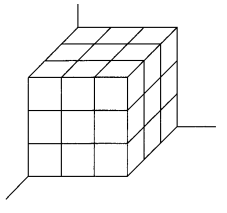Volume = _________ cubic units
Volume of give cube has 27 cubic units,

Explanation:
As we know volume of  solid is l X w X h,
so given cube  has 3 unit X 3 unit X 3 unit = 27 cubic units.

Question 2.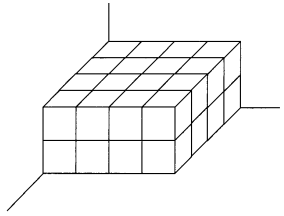Volume = _________ cubic units
Volume of give cube has 32 cubic units,

Explanation:
As we know the volume of solid is l X w X h,
so the given cube has 4 units X 4 units X 2 units = 32 cubic units.

Question 3.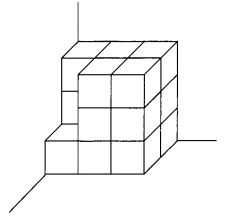Volume = _________ cubic units
Volume of given cube has  16 cubic units,

Explanation:
As we know the volume of  solid is l X w X h,
Given cube contains 2 fewer small unit cubes so first we
calculate the total surface and subtract missing cubic units,
Total surface area has 3 units X 2 units X 3 units = 18 cubic units.
the surface area of small unit cubes is 1 unit X 1 unit X 2 units = 2 cubic units,
therefore the volume of the given cube is 18 cubic units – 2 cubic units = 16 cubic units.

Question 4.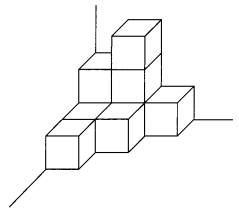Volume = _________ cubic units
Volume of 9 cubic units,

Explanation:
Given solid cube has 9 unit cubes with 1 unit X 1 unit X 1 unit each,
So the volume of given cube is 9 X (1 unit X 1 unit X 1 unit)  = 9 cubic units.

These solids are formed by stacking 1-centimeter cubes in the corner of a room.
Find the volume of each solid.

Question 5.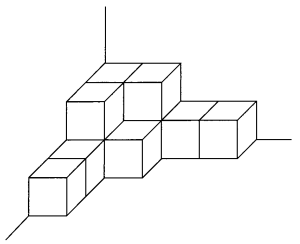Volume = ______ cm3
Volume 11 cm3,

Explanation:
Given solid cube has 11 units cms with 1 cm X 1 cm X 1 cm each,
So the volume of given cubes is 11 X (1 cm X 1 cm X 1 cm)  = 11 cm3.

Question 6.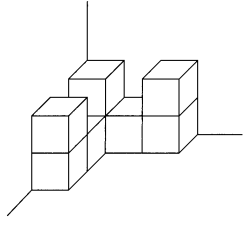Volume = _________ cm3
Volume 8 cm3,

Explanation:
Given solid cube has 8 units cms with 1 cm X 1 cm X 1 cm each,
So the volume of given cubes is 8 X (1 cm X 1 cm X 1 cm)  = 8 cm3.

Question 7.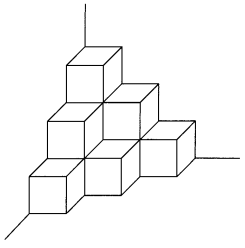Volume = _________ cm3
Volume 10 cm3,

Explanation:
Given solid cube has 10 units cms with 1 cm X 1 cm X 1 cm each,
So the volume of given cubes is 10 X (1 cm X 1 cm X 1 cm)  = 10 cm3.

Question 8.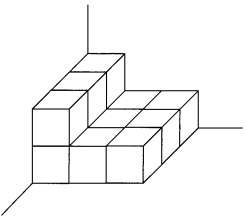Volume = ______ cm3
Volume 12 cm3,

Explanation:
Given solid cube has 12 units cms with 1 cm X 1 cm X 1 cm each,
So the volume of given cubes is 12 X (1 cm X 1 cm X 1 cm)  = 12 cm3.

Question 9.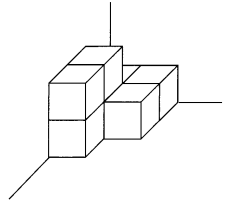Volume = _________ cm3
Volume 7 cm3,

Explanation:
Given solid cube has 7 units cms with 1 cm X 1 cm X 1 cm each,
So the volume of given cubes is 7 X (1 cm X 1 cm X 1 cm)  = 7 cm3.

Question 10.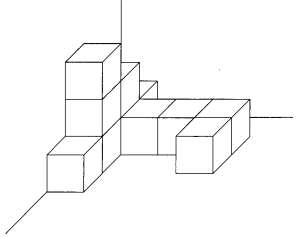Volume = _________ cm3
Volume 11 cm3,

Explanation:
Given solid cube has 11 units cms with 1 cm X 1 cm X 1 cm each,
So the volume of given cubes is 11 X (1 cm X 1 cm X 1 cm)  = 11 cm3.

These solids are built using 1-centimeter cubes. Find the volume of each solid.
Then compare their volumes and fill in the blanks.

Question 11.Solid _________ has a greater volume than solid _________.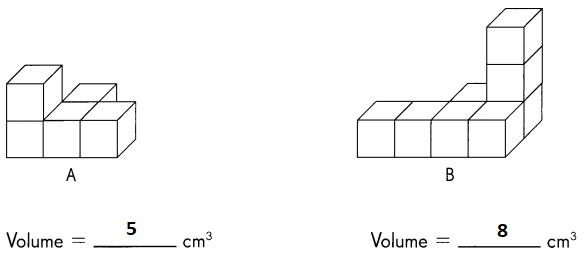Solid B has a greater volume than solid A,

Explanation:
Given solid cube A has 5 units cms with 1 cm X 1 cm X 1 cm each,
So the volume of given cubes is 5 X (1 cm X 1 cm X 1 cm)  = 5 cm3,
and given solid cube B has 8 units cms with 1 cm X 1 cm X 1 cm each,
So the volume of given cubes is 8 X (1 cm X 1 cm X 1 cm)  = 8 cm3.
Solid B has a greater volume than solid A.

These solids are built using 1-meter cubes. Find the volume of each solid.
Then compare their volumes and fill in the blanks.

Question 12.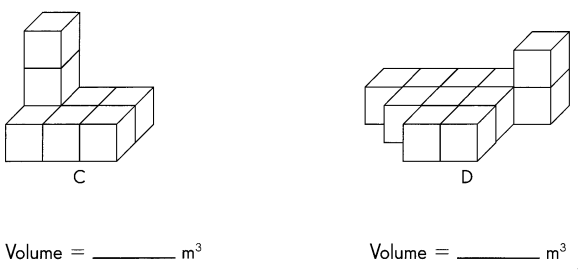Solid _________ has less volume than solid _________.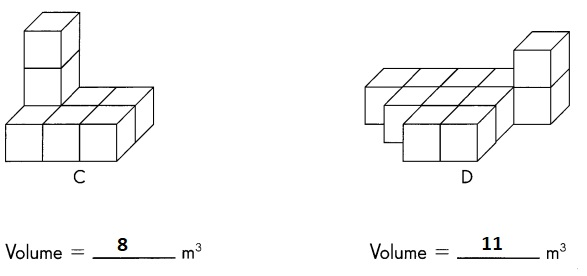Solid 8 m3 has less volume that solid 11 m3,

Explanation:
Given solid cube C has 8 units m’s with 1 m X 1 m X 1 m each,
So the volume of given cubes is 8 X (1 m X 1 m X 1 m)  = 8 m3,
and given solid cube D has 11 units m’s with 1 m X 1 m X 1 m each,
So the volume of given cubes is 11 X (1 m X 1 m X 1 m)  = 11 m3.
Solid 8 m3 has less volume that solid 11 m3.

These solids are built using 1-inch cubes.
Find the volume of each solid.
Then compare their volumes and f411 in the blanks.

Question 13.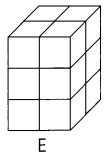Length = __________ in.
Width = __________ in.
Height = _________ in.
Volume = _________ in.3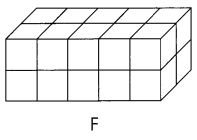Length = __________ in.
Width = __________ in.
Height = _________ in.
Volume = _________ in.3
Solid _________ has less volume than solid _________.
Solid E has less volume than solid F,

Explanation:
Given the solid cube of E which has a length of 2 in, width 2 in and height 3 in,
so the volume of cube E is 12 in.3,
Given solid cube of F which has a length of 4 in, width of 2 in, and height of 2 in,
so volume of cube F is 16 in.3.
Solid E has less volume than solid F.

These solids are built using 1-foot cubes. Find the volumes of each solid.
Then compare their volumes and fill in the blanks.

Question 14.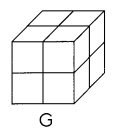Length = _____2_____ ft.
Width = ______2____ ft.
Height = _____2____ ft.
Volume = _____8____ ft.3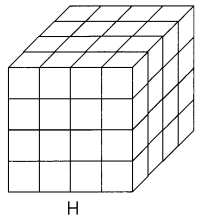Length = ____4______ ft.
Width = _____4_____ ft.
Height = _____4____ ft.
Volume = _____64____ ft.3
Solid _________ has a greater volume than solid _________.